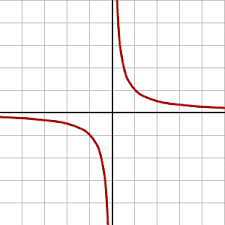# Calculating Maximum Height: Confusion & Solutions

• annamal

#### annamal

Homework Statement
What is the minimum height ℎ0 above ground that is required to generate the greatest range shooting horizontally with an angle of 0 degrees and discounting air resistance? 𝑣0 is initial velocity.
Relevant Equations
y = h + vt + 0.5a*t^2
vx = v0*cos(theta)
vy = v0*sin(theta) - g*t
I am calculating it like this:
𝑦=ℎ0−0.5𝑔𝑡^2=0→ℎ0=0.5𝑔𝑡^2→𝑡=sqrt(2*ℎ0/g)

𝑥=𝑣0*𝑡→ substituting t →𝑥=𝑣0*sqrt(2*ℎ0/g)

𝑑𝑥/𝑑ℎ0=𝑣0/(𝑔*sqrt(2*h0/g))=0 for maximum ℎ0=0.
confused. can someone tell me how I am calculating this wrong?

•berkeman
The question doesn't seem to make much sense.

eg: "angle of 0 degrees".

Last edited:
Homework Statement:: What is the minimum height ℎ0 above ground that is required to generate the greatest range shooting horizontally with an angle of 0 degrees and discounting air resistance?
Uh ... as high as you can get. You don't see how that question makes no sense?

𝑑𝑥/𝑑ℎ0=𝑣0/(𝑔*sqrt(2*h0/g))=0 for maximum ℎ0=0.
confused. can someone tell me how I am calculating this wrong?
Does ##dx/dh_0=0## necessarily give you the point where x reaches a maximum? In what two ways might that not be true.

𝑑𝑥/𝑑ℎ0=𝑣0/(𝑔*sqrt(2*h0/g))=0 for maximum ℎ0=0.
So you have this formula for the first derivative of range as a function of height. And you seem have observed that this first derivative is maximized when height ##h_0## approaches zero.

But you seem to have lost track of what you were doing. You are looking for a height that makes the first derivative zero. Not a height that maximizes it.

If you plotted the first derivative, you could see that it looks a bit like just the first quadrant of a hyperbola.